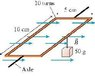# Torque on Current Loops

• dtesselstrom
In summary, the problem is that the person is not sure what the equation should be for the existing numbers. They have tried all sorts of things, but they haven't been able to find a solution.f

## Homework Statement

The 10-turn loop of wire shown in the figurelies in a horizontal plane, parallel to a uniform horizontal magnetic field, and carries a 2.0 A current. The loop is free to rotate about a nonmagnetic axle through the center. A 50 g mass hangs from one edge of the loop.

Torque = u * B
Torque = (I*A) B

## The Attempt at a Solution

Ive tried all sorts of things I konw that I is 2.0 A and A is .1 * .05 so that gives .01 * B = Torque and I've set torque equal to just the F of the weight times the radius and diameter and I've also tried doubling the F because the force down on both sides. No matter what I've tried it doesn't seem to work. Answers that I have gotten are 1.225. 2.45, 4.9, and .6125 so if you try this problem and get those they are wrong. Thanks for the help.

#### Attachments

•knight_Figure_32_80.jpg
12 KB · Views: 458
If there are 10 turns in the loop, wouldn't you use the equation that incorporates the # of turns?

As far as I know, the 2nd equation you stated holds true for rectangular loops (I can't see your attachment so I don't really know what the problem set up is).

Additionally, if the loops is parallel to the magnetic field...what would sin(theta) be?

Last edited:
I can't find any equations in my book for turns in the loop so if you could help me out with that Id appriciated it and I was wondering that myself as for the picture it is set up as a rectangular loop so I wasn't sure that the turns ment anything and wouldn't it make the sin of theta 90 degrees?

For any other loop that is not a rectangle, torque=NIABsin(theta), where N=# of turns.

ok got it thanks for the help.

ok got it thanks for the help.[/QUO
What is the answer for this question for the existing numbers? Can you help?

Did anybody come up with an answer for this question?